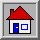#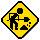Hard hat area, construction in progress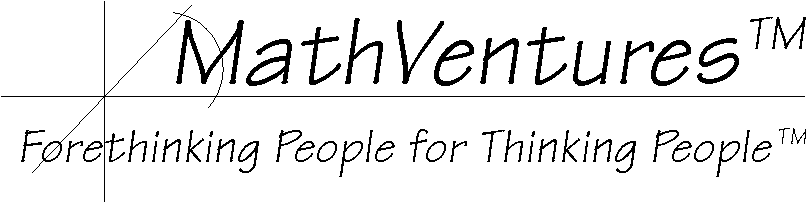# Logic  the Art of Reasoning

Mathematics   the Art of Studying Patterns Using Logic

# The Numbers`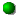The Basic Counting Numbers     formally called the natural numbers or the positive integers.`
`The counting-in-place number   formally known as Zero.`
`The counting-backward numbers or counting-down numbers  adding the negative integers, together we have the integers.`
```The Expanding Counting Numbers, that is, numbers for counting up and down or forward, backward and in place or sideways.
Formally called the integers, these numbers consist of the natural numbers, zero and negative integers. They appear in discrete, fixed interval.```
`The Measuring Numbers  adding the fractions, together we have the rational numbers.`
`Estimating Numbers     adding the irrational numbers, together we have the real numbers.`
`Spatial Numbers        adding the imaginary numbers, together we have the complex numbers.`
```These numbers include all the previous number and then they add the imaginary numbers,
which are the result of taking the square root of the negative numbers.```
```The imaginary numbers exist in the plane. That is, an imaginary number does not lie on the number line
but rather in the two-dimensional space.```
``

# The Numbers'  Tree

`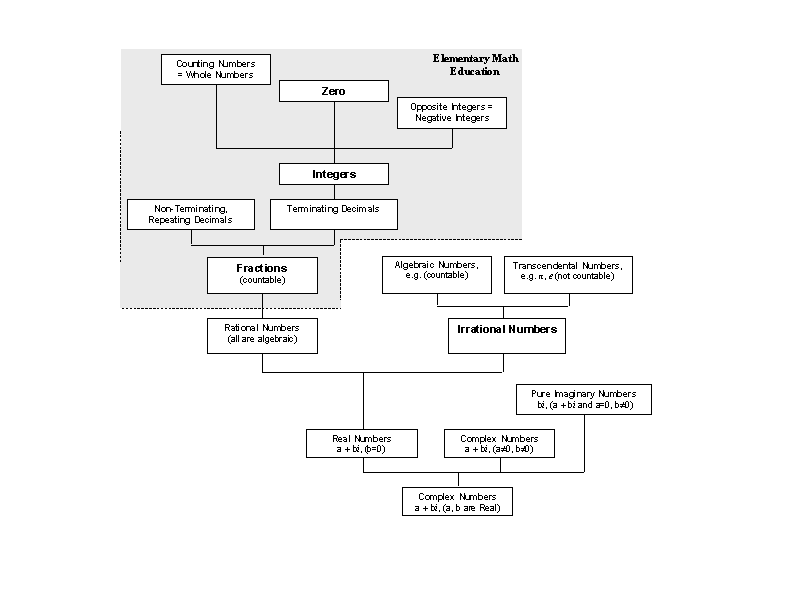`

# The Numbers'  Venn Diagram

`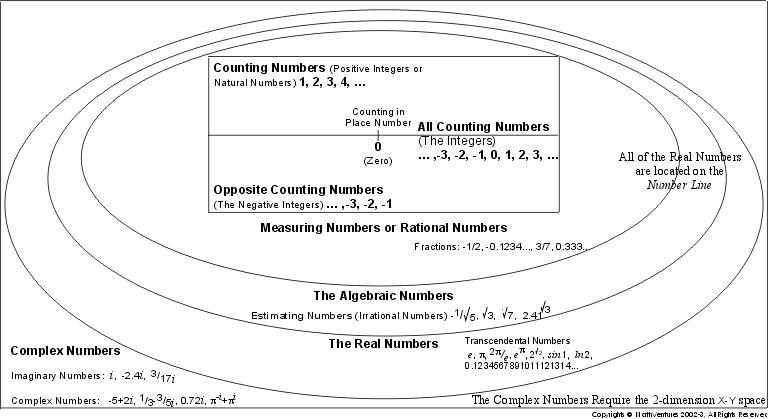`